# Linear, quadratic, cubic equations, roots of equations. Algebra IIM CAT, XAT Quant sample Questions : CAT, MBA Entrance Test Questions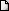LATEST NEWS • Ascent Success Stories • TANCET 16 Classes @ Chennai • XAT 16 Classes @ Chennai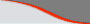Home | TANCET Coaching Class | TANCET study material | Online TANCET Course | Contact us
 Ascent's Courses TANCET Coaching Chennai Online TANCET Course TANCET MBA Study Material CAT Classroom Chennai CAT Correspondence India Mock CAT Series GRE Coaching Chennai GMAT Coaching Chennai Online GMAT Course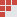US B Schools Faculty List Jobs @ Ascent Testimonials Ascent Success Stories Contact Us +91 44 4500 8484 +91 96000 48484
You are here: Home » CAT Math » Algebra » Co-efficient of quadratic equation

#### Quadratic Equation - CAT 2016 Math Preparation

Question 4 the day : March 2, 2004
The question for the day is a sample practice problem in quadratic equation. Helps understand concepts related to roots of a quadratic equation and the nature of the roots of the quadratic equation. The question for the day is on finding a missing co-efficient from the topic quadratic equation..

Question
If one of the roots of the quadratic equation x2 + mx + 24 = 0 is 1.5, then what is the value of m?
1. -22.5
2. 16
3. -10.5
4. -17.5
Correct Answer is -17.5. Correct Choice is (4)

We know that the product of the roots of a quadratic equation ax2 + bx + c = 0 is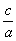In the given equation, x2 + mx + 24 = 0, the product of the roots =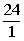= 24.
The question states that one of the roots of this equation = 1.5.
If x1 and x2 are the roots of the given quadratic equation and let x1 = 1.5.
Therefore, x2 == 16.
In the given equation, m is the co-efficient of the x term.
We know that the sum of the roots of the quadratic equation ax2 + bx + c = 0 is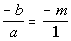= -m
Sum of the roots = 16 + 1. 5 = 17 = -17.5.
Therefore, the value of m = -17.5

## More Questions on Linear, Quadratic and Cubic Equations - Algebra

 5. 6. 7.

## XLRI XAT TANCET Practice Question Samples

 TANCET MBA MCA Practice Questions with detailed explanation and shortcuts.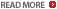Comprehensive study material for GMAT Quant. 1800+ questions.Ascent offers CAT Correspondence Course for those who reside abroad.